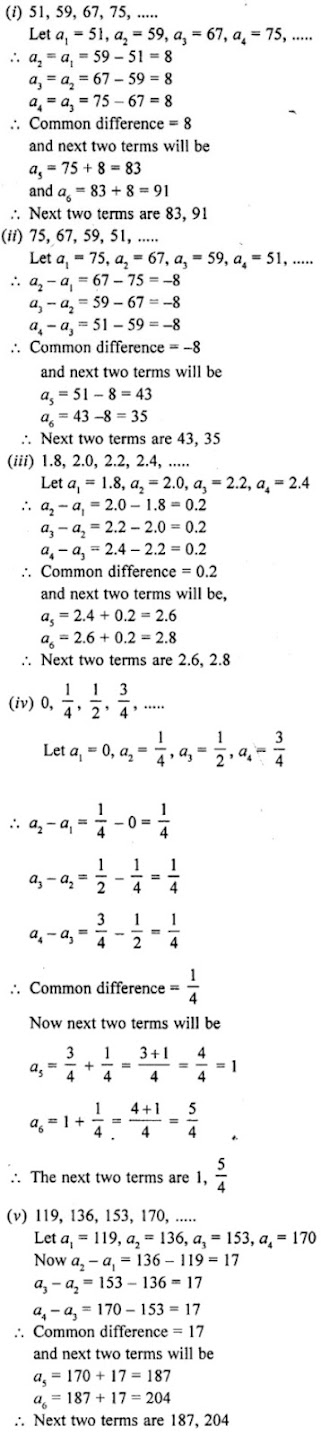# RD Sharma Solutions for Class 10 Maths Chapter 9 Arithmetic Progression Exercise 9.3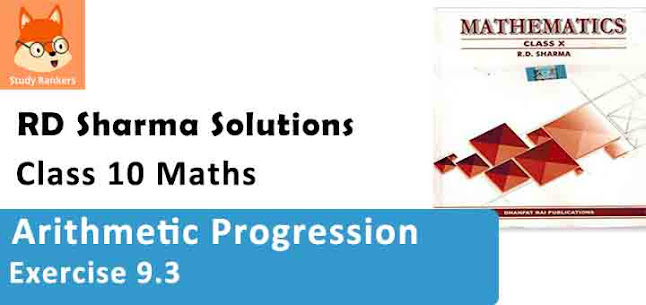1. For the following arithmetic progressions write the first term a and the common difference d :

(i) -5, -1, 3, 7, ……
(ii) 1/5 , 3/5 , 5/5 , 7/5 , ……
(iii) 0.3, 0.55, 0.80, 1.05, ……
(iv) -1.1, -3.1, -5.1, -7.1, ……

Solution

(i) -5, -1, 3, 7, ……
Here, first term (a) = -5
We have, a2 - a1 = -1 - (-5) = 4
a3 - a2 = 3 - (-1) = 3 + 1 = 4
a4 - a3 = 7 - 3 = 4
∴ Common difference (d) = 4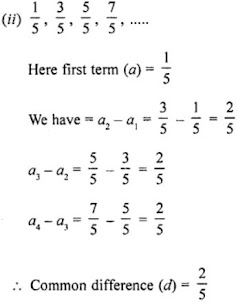(iii) 0.3, 0.55, 0.80, 1.05,....
Here, first term (a) = 0.3
We have = a2- a1 = 0.55 - 0.3 = 0.25
a3 - a2 = 0.80 - 0.55 = 0.25
∴ Common difference = 0.25

(iv) -1.1, -3.1, -5.1, -7.1,....
Here, first term (a) = -1.1
We have = a2 - a1 = -3.1 - (-1.1)
= -3.1 + 1.1 = -2.0
a3 - a2 = -5.1 - (-3.1) = -5.1 + 3.1 = -2.0
a4 - a3 = -7.1 - (-5.1) = -7.1 + 5.1 = -2.0
∴ Common difference = -2.0

2. Write the arithmetic progression when first term a and common difference d are as follows:

(i) a = 4, d = -3
(ii) a = -1, d= 12
(iii) a = -1.5, d = -0.5

Solution

(i) First term (a) = 4
and common difference (d) = -3
Second term = a + d = 4 – 3 = 1
Third term = a + 2d = 4 + 2×(-3) = 4 – 6 = -2
Fourth term = a + 3d = 4 + 3 (-3) = 4 – 9 = -5
Fifth term = a + 4d = 4 + 4 (-3) = 4 – 12 = -8
AP will be 4, 1, -2, -5, -8, ….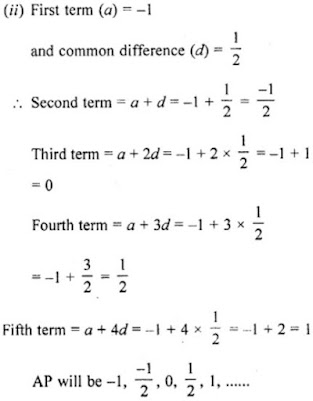(iii) First term (a) = -1.5 and common difference (d) = -0.5
Second term = a + d = -1.5 + (-0.5)
= -1.5 - 0.5 = -2.0
Third term = a + 2d = -1.5 + 2×(-0.5)
= -1.5 - 1.0 = -2.5
Fourth term = a + 3d = -1.5 + 3×(-0.5)
= 1.5 - 1.5 = -3.0
Fifth term = a + 4d = -1.5 + 4×(-0.5)
= -1.5 - 2.0 = -3.5
AP will be -1.5, -2.0, -2.5, -3.0, -3.5,....

3. In which of the following situations, the sequence of numbers formed will form an A.P?
(i) The cost of digging a well for the first metre is ₹ 150 and rises by ₹ 20 for each succeeding metre.
(ii) The amount of air present in the cylinder when a vacuum pump removes each time 1/4 of the remaining in the cylinder.
(iii) Divya deposited ₹ 1000 at compound interest at the rate of 10% per annum. The amount at the end of first year, second year, third year, …, and so on. [NCERT Exemplar]

Solution

(i) Cost of digging a well for the first metre = ₹ 150
Cost for the second metre = ₹ 150 + ₹ 20 = ₹ 170
Cost for the third metre = ₹ 170 + ₹ 20 = ₹ 190
Cost for the fourth metre = ₹ 190 + ₹ 20 = ₹ 210
The sequence will be (In rupees)
150, 170, 190, 210, ……
Which is an A.P.
Whose = 150 and d = 20

(ii) Let air present in the cylinder = 1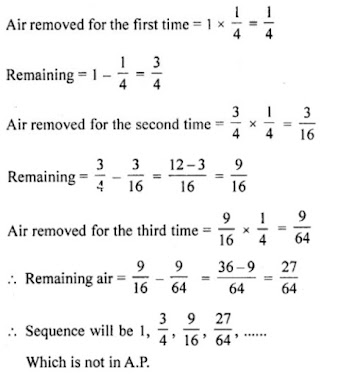(iii) Amount at the end of the 1st year = ₹ 1100
Amount at the end of the 2nd year = ₹ 1210
Amount at the end of 3rd year = ₹ 1331 and so on.
So, the amount (in ₹) at the end of 1st year, 2nd year, 3rd year, … are
1100, 1210, 1331, …….
Here, a2 – a1 = 110
a3 – a2 = 121
As, a2 – a1 ≠ a3 – a2, it does not form an A.P.

4. Find the common difference and write the next four terms of each of the following arithmetic progressions :

(i) 1, -2, -5, -8, ……
(ii) 0, -3, -6, -9, ….
(iii) -1, 1/4 , 3/2 , ….
(iv) -1, – 5/6 , – 2/3 , ….

Solution

(i) 1, -2, -5, -8,....
Here, a1 = 1, a2 = -2, a3 = -5, a4 = -8,....
Now a2 - a1 = -2-1 = -3
a3 - a2 = -5-(-2) = -5 + 2 = -3
a4 - a3 = -8-(-5) = -8 + 5 = -3
It is an A.P. whose common difference is = -3
Now, next four terms will be
-8 - 3 = -11
-11 - 3 = -14
-14 - 3 = -17
-17 - 3 = -20
∴ -11, -14, -17, -20 are four term next to these.

(ii) 0, -3, -6, -9,....
Here, a1 = 0, a2 = -3, a3= -6, a4 = -9
Now, a2 - a1 = -3 - 0 = -3
a3 - a2 = -6-(-3) = -6 + 3 = -3
a4 - a3 = -9-(-6) = -9 + 6 = -3
We see that it is an A.P. whose common difference is -3.
Now, next four terms will be
-9 - 3 = -12
-12 - 3 = -15
-15 - 3 = -18
-18 - 3 = -21
∴ -12, -15, -18, -21 are next four terms.5. Prove that no matter what the real numbers a and b are, the sequence with nth term a + nb is always an A.P. What is the common difference ?

Solution

an = a + nb
Let n= 1, 2, 3, 4, 5, ……….
a1 = a + b
a2 = a + 2b
a3 = a + 3b
a4 = a + 4b
a5 = a + 5b
We see that it is an A.P. whose common difference is b and a for any real value of a and b as
a2 – a1 = a + 2b – a – b = b
a3 – a2 = a + 3b – a – 2b = b
a4 – a3 = a + 4b – a – 3b = b
and a5 – a4 = a + 5b – a – 4b = b

6. Find out which of the following sequences are arithmetic progressions. For those which are arithmetic progressions, find out the common difference.

(i) 3, 6, 12, 24,....
(ii) 0, -4, -8, -12,....
(iii) 1/2, 1/4, 1/6, 1/8,....
(iv) 12, 2, -8, -18,....
(v) 3, 3, 3, 3,....
(vi) p, p + 90, p + 180, p + 270,.... where p = (999)999
(vii) 1.0, 1.7, 2.4, 3.1,....
(viii) -225, -425, -625, -825,....
(ix) 10, 10 + 25, 10 + 26, 10 + 27,....
(x) a+b, (a+ 1)+b, (a+ 1)+(b+1), (a+ 2) + (b + 1), (a +2) + (b + 2),....
(xi) 12, 32, 52, 72,....
(xii) 12, 52, 72, 73,....

Solution

(i) 3, 6, 12, 24,....
Let a1 = 3, a2 = 6, a3 = 12, a4 = 24
Now, a2 - a1 = 6 - 3 = 3
a3 - a2 = 12 - 6 = 6
a4 - a3 = 24- 12 = 12
It has no common difference.
∴ It is not an A.P.

(ii) 0, -4, -8, -12,....
Let a1 = 0, a2 = -4, a3 = -8, a4 = -12
Now, a2 - a1 = -4 - 0 = -4
a3 - a2 = -8 - (-4) = -8 + 4 = -4
a4 - a3 = -12 - (-8) = -12 + 8 = -4
Here, we see that common difference is -4.
∴ It is an A.P.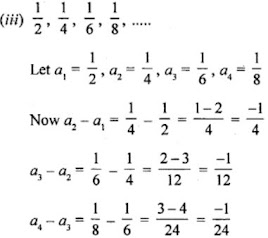We see that there is no common difference.
It is not an A.P.

(iv) 12, 2, -8, -18,....
Let a1 = 12, a2 = 2, a3 = -8, a4 = -18
Now, a2 - a1 = 2 - 12 = -10
a3 - a2 = -8 - 2 = -10
a4 - a3 = -18 -(-8) = -18 + 8 = -10
We see that common difference is -10.
∴ It is an A.P.

(v) 3, 3, 3, 3,....
Let a1 = 3, a2 = 3, a3 = 3, a4 = 3,....
Now, a2 - a1 = 3 - 3 = 0
a3 - a2 = 3 - 3 = 0
a4 - a3 = 3 - 3 = 0
We see that common difference is 0.
∴ It is an A.P.

(vi) p, p + 90, p + 180, p + 270,.... where p = (999)999
Let a1 = p, a2 = p + 90, a3 = p + 180, a4 = p + 270
Now,
a2 - a1 = p + 90 - p = 90
a3 - a2 = p + 180 - p - 90 = 90
a4 - a3 = p + 270 - p - 180 = 90
We see that common difference is 90.
∴ It is an A.P.

(vii) 1.0, 1.7, 2.4, 3.1,....
Let a1 = 1.0, a2 = 1.7, a3 = 2.4, a4 = 3.1
Now a2 - a1 = 1.7- 1.0 = 0.7
a3 - a2 = 2.4 - 1.7 = 0.7
a4 - a3 = 3.1 - 2.4 = 0.7
We see that common difference is 0.7.
∴ It is an A.P.

(viii) -225, -425, -625, -825,....
Let a1 = -225, a2 = -425, a3 = -625, a4 = -825
Now, a2 - a1 = -425 -(-225) = -425 + 225 = -200
a3 - a2 = -625 -(-425) = -625 + 425 = -200
a4 - a3 = -825 -(-625) = -825 + 625 = -200
We see that common difference is -200.
∴ It is an A.P.

(ix) 10, 10 + 25, 10 + 26, 10 + 27,....
Let a1 = 10, a2 = 10 + 25, a3 = 10 + 26, a4 = 10 + 27
Now, a2 - a1 = 10 + 25 - 10 = 25 = 32
a3 - a2 = 10 + 26 - 10 - 25 = 26- 25 = 64 - 32 = 32
a4 - a3 = 10 + 27 - 10 - 26 = 27- 26 = 128 - 64 = 64
We see that there is no common difference.
∴ It is not an A.P.

(x) a+b, (a+1)+b, (a+1)+(b+1), (a+2)+(b+1), (a+2)+(b+2),....
Let a1 = a+b
a2= (a+1)+b
a3 = (a+1)+(b+1)
a4 = (a+2)+(b+1)
a5 = (a+2)+(b+2)
Now, a2 - a1 = (a+1)+b-a-b = a+1+b-a-b = 1
a3- a2 = (a+1)+(b+1)-{(a+1)+b} = a+1+b+1-a-1-b = 1
a4 - a3 = {(a+2)+(b+1)}-{(a+1)+(b+1)) = a+2+b+1-a-1-b-1 = 1
a5- a4 = {(a+2)+(b+2)} - {(a+2)+(b+1)} = a+2+b+2-a-2-b-1 = 1
We see that common difference is 1.
∴ It is an A.P.

(xi) 12, 32, 52, 72,...
Let a1 = 12, a2 = 32, a3= 52, a4= 72
Now, a2 - a1 = 32 - 12 = 9-1 = 8
a3 - a2 = 52 - 32 = 25 - 9 = 16
a4 - a3 = 72 - 52 = 49 - 25 = 24
We see that there is no common difference.
∴ It is not an A.P.

(xii) 12, 52, 72, 73,....
Let a1 = 12, a2 = 52, a3 = 72, a, = 73
Now, a2 - a1 = 52 - 12 = 25 - 1 =24
a3 - a2 = 72 - 52 = 49 - 25 = 24
a4 - a3 = 73 - 72 = 73 - 49 = 24
We see that the common difference is 24.
∴ It is an A.P.

7. Find the common difference of the A.P. and write the next two terms :

(i) 51, 59, 67, 75, …….
(ii) 75, 67, 59, 51, ……
(iii) 1.8, 2.0, 2.2, 2.4, ……
(iv) 0, 1/4 , 1/2 , 3/4 , ……
(v) 119, 136, 153, 170, ……

Solution